• 绘制整个语音的短时能量和一帧的短时能量对比 import numpy as np import wave import matplotlib.pyplot as plt wlen = 512 inc = 128 f = wave.open('./data/orange/orange06.wav', 'rb') params = f.getparams()...
绘制整个语音的短时能量和一帧的短时能量对比
import numpy as np
import wave
import matplotlib.pyplot as plt

wlen = 512
inc = 128
f = wave.open('./data/orange/orange06.wav', 'rb')
params = f.getparams()
nchannels, sampwidth, framerate, nframes = params[:4]

wave_data = np.fromstring(str_data, dtype=np.short)
wave_data = wave_data * 1.0 / (max(abs(wave_data)))
time = np.arange(0,wlen) * (1.0 / framerate)
signal_length = len(wave_data)

if signal_length<=wlen:
nf = 1
else:
nf = int(np.ceil((1.0 * signal_length - wlen + inc) / inc))
# 所有帧加起来总的铺平后的长度
pad_length = int((nf - 1) * inc + wlen)
# 不够的长度使用0填补，类似于FFT中的扩充数组操作
# 相当于对所有帧的时间点进行抽取，得到nf*nw长度的矩阵
indices = np.tile(np.arange(0, wlen), (nf, 1)) + np.tile(np.arange(0, nf * inc, inc),
(wlen, 1)).T
# 将indices转化为矩阵
indices = np.array(indices, dtype=np.int32)
# 得到帧信号
# 读取第30-31这一帧
a=frames[30:31]
# 一帧短时能量的x轴配置
time_0 = np.arange(0, wlen) * (1.0 / framerate)
# 整个语音段的短时能量x轴配置
time_all = np.arange(0, nf) * (inc * 1.0 /framerate)
# 添加汉明窗
windown = np.hanning(512)
d = np.zeros(nf)
x = np.zeros(nf)
# 绘制整个语音段的短时能量
for i in range(0, nf):
a = frames[i:i+1]
b = a * windown
c = np.square(b)
d[i] = np.sum(c)
d = d*1.0/max(abs(d))
# 一帧的短时能量
k = a*windown
m = np.square(k)

# All short-time energy
plt.figure(figsize=(10, 4))
plt.subplot(3, 1, 1)
plt.plot(time_all, d, c="g")
plt.xlabel('All short-time energy')
plt.grid()
# Frame short-time energy
plt.subplot(3, 1, 3)
plt.plot(time_0, m, c='b')
plt.xlabel('Frame short-time energy')
plt.grid()
# show
plt.show()​											发音为apple的短时能量图
短时平均过零率
短时平均过零率-表示一帧语音中语音信号波形穿过横轴（零电平）的次数。对于连续语音信号，过零意味着时域波形通过时间轴，对于离散信号，如果相邻的取样值改变符号，则称为过零。短时平均过零率就是样本数值改变符号的次数。
浊音具有较低的过零率，而清音具有较高的过零率。在背景噪声较小时，用平均能量识别较为有效；在背景噪声较大时，用短时平均过零率较为有效。
主要应用短时平均过零率来判断清音和浊音，有话段和无话段。
# 绘制过零率
c_zero = np.zeros(nf)
for i in range(nf):
a_zero = frames[i:i+1]
b_zero = windown * a_zero
for j in range(wlen-1):
if b_zero[j] * b_zero[j+1] < 0:
c_zero[i] = c_zero[i] + 1
time = np.arange(0,nf) * (inc*1.0/framerate)
plt.figure(figsize=(10,4))
plt.plot(time,c,c="g")
plt.grid()
plt.show()时域和频域的转换
短时傅里叶分析适用于分析缓慢时变信号的频谱分析。先将语音信号分帧，再将各帧进行傅里叶变换，每一帧语音信号可以被认为是从各个不同的平稳信号波形中截取出来的，各帧语音的短时频谱就是各个平稳信号波形频谱的近似。
而语音信号是短时平稳的，因此可以对语音信号进行分帧处理。
"""频域处理，时域转换为频域"""
import numpy as np
import wave
import matplotlib.pyplot as plt

wlen = 512
inc = 128
f = wave.open('./data/apple/apple02.wav', 'rb')
params = f.getparams()
nchannels, samwidth, framerate, nframes = params[:4]
wave_data = np.fromstring(str_data, dtype=np.short)
wave_data = wave_data * 1.0 / (max(abs(wave_data)))
# 信号总长度
signal_length = len(wave_data)
if signal_length<=wlen:
nf = 1
else:
nf = int(np.ceil((1.0*signal_length-wlen+inc)/inc))
# 所有帧加起来的总长度
# 0 填充
indices = np.tile(np.arange(0,wlen),(nf,1))+np.tile(np.arange(0,nf*inc,inc),(wlen,1)).T
indices = np.array(indices, dtype=np.int32)
windown = np.hanning(wlen)

a = frames[20:21]
b = a * windown
# 短时傅里叶变换
fft_signal_short = np.fft.fft(b)
# 取变换的模
fft_signal_short = abs(fft_signal_short)
# 傅里叶变换
fft_signal = np.fft.fft(wave_data)
#  取变换的模
fft_signal = abs(fft_signal)

plt.figure(figsize=(10, 4))
time = np.arange(0, nframes) * framerate / nframes
time_short = np.arange(0, wlen) * framerate / nframes
plt.subplot(3, 1, 1)
plt.plot(time, fft_signal, c='b')
plt.xlabel('FFT')
plt.grid()
plt.subplot(3, 1, 3)
plt.plot(time_short, fft_signal_short, c='g')
plt.xlabel('STFT')
plt.grid()
plt.show()语谱图
plt.specgram(wave_data,Fs = framerate, scale_by_freq = True, sides = 'default')

语谱图的横坐标是时间，纵坐标是频率，坐标点值为语音数据能量。由于是采用二维平面表达三维信息，所以能量值的大小是通过颜色来表示，颜色深表示该点的语音能量强。


展开全文可视化 语音识别 自然语言处理
• 也就是说，一帧时间内的语音能量就是短时能量，语音的短时能量就是将语音中每一帧的短时能量都计算出来，然后我们就可以利用这个短时能量数组做很多事情了。通常而言，短时能量用途有以下几个方面：
介绍
刚开始学习计算机视听觉，第一个实验是端点检测算法。这个算法实现起来还是比较简单的，主要是该算法利用到的两个数据——语音短时能量和短时过零率。今天先分享一下我计算短时能量的方法。
语音短时能量，顾名思义就是计算较短时间内的语音能量。这里的较短时间，通常指的是一帧。也就是说，一帧时间内的语音能量就是短时能量，语音的短时能量就是将语音中每一帧的短时能量都计算出来，然后我们就可以利用这个短时能量数组做很多事情了。通常而言，短时能量用途有以下几个方面：

区分清音和浊音
判定有声段和无声段
对声母和韵母分界
可以作为辅助的特征参数用于语音识别中，经常是识别系统中的特征一维

端点检测利用的就是短时能量的前两个用途——将语音文件中的语音分离出来。
介绍完定义后，再来看看短时能量的计算公式。
计算
语音能量的计算，通常是 $E ={x(n)}^2$。由语音短时能量的定义，就可以得到短时能量的计算公式：
$E_n = \sum^\infty_{m = -\infty}[x(m)w(n - m)]^2 = {x(n)}^2 * h(n)$
其中，$h(n) = {w(n)}^2$ ， $w(n)$ 是窗口函数
也可以简写成：
$E_n = \sum^\infty_{m = -\infty}[x(m)w(m)]^2$
由于短时能量是语音的时域特征，因此，在不使用傅里叶变换的情况下，这里的窗口是一种方窗，即：
$w(n)= \begin{cases} 1& \text {0 <= n <= N - 1} \\ 0 & \text{其它} \end{cases}$
因此，可以得出，这里的语音短时能量就相当于，每一帧中所有语音信号的平方和。
Python 实现短时能量
首先读取语音文件，我这里是 .wav 类型的，不同类型可能文件格式不一样，我们只需要读取到语音的采样点部分就可以了。
f = wave.open("./语料/" + str(i + 1) + ".wav","rb")
# getparams() 一次性返回所有的WAV文件的格式信息
params = f.getparams()
# nframes 采样点数目
nchannels, sampwidth, framerate, nframes = params[:4]
str_data = f.readframes(nframes)            # str_data 是二进制字符串

# 转成二字节数组形式（每个采样点占两个字节）
wave_data = np.fromstring(str_data, dtype = np.short)
print( "采样点数目：" + str(len(wave_data)))          #输出应为采样点数目
f.close()

计算语音短时能量函数如下：
# 计算每一帧的能量 256个采样点为一帧
def calEnergy(wave_data) :
energy = []
sum = 0
for i in range(len(wave_data)) :
sum = sum + (int(wave_data[i]) * int(wave_data[i]))
if (i + 1) % 256 == 0 :
energy.append(sum)
sum = 0
elif i == len(wave_data) - 1 :
energy.append(sum)
return energy

最后直接调用：
energy = calEnergy(wave_data)

总结
最后，语音短时能量是一个比较简单的语音时域特征，也是一个语音学中经常使用的特征，希望这篇文章能够帮助大家。
由于本人刚开始学习语音部分，学的还很有限，如有书写错误的地方，希望大家帮助改正，谢谢。


展开全文语音信号处理
• 特征提取（Feature Exaction）的重要性，就不用多说了。对于音频信号，按时间分辨率、按局部or全局的观念、持续...短时能量、功率是有点瞬时功率的概念，但又没有那么“瞬时”。大概是按帧在做计算。短时功率在计...
特征提取（Feature Exaction）的重要性，就不用多说了。对于音频信号，按时间分辨率、按局部or全局的观念、持续时间长短，或者爱怎么讲怎么讲，特征可分为长期（long-term）、中期（mid-term）、短期（short-term），也可以叫短时特征。术语翻译不统一，我也不专业。知道英文术语就好。短时能量、功率是有点瞬时功率的概念，但又没有那么“瞬时”。大概是按帧在做计算。短时功率在计算的时候，把每一帧的能量还要处理该帧的长度，量纲上等于[Watt]。有时候，要把线性尺度的短时能量和短时功率换算成对数尺度，分贝（dB），还要对最大值进行归一化，换算成相对强度，让最大值为0dB。短时过零率（short-term zero corss rate，st-ZCR），大概是从时域上描述信号频率吧，也是按帧计算。声母的ZCR高一些，韵母的要低一些。噪声的话，不太确定了。大概好像要是比韵母高。和声母，我还不太清楚。大致的代码如下了。短时能量。function E = get_st_energy( x,fs,wlen_time,step_time,win_type,energy_unit )
%function zcr = get_st_energy( x,fs,wlen_time,step_time,win_type,energy_unit )
%   获取短时能量（没有除以帧长，所以不是计算的功率）。
%   输入参数
%           x：语音信号 --> 单声道
%           fs：采样速率
%           wlen_time：窗口时间（s）
%           step_time：步进时间（s）
%           win_type：'hamming','hanning',...,默认'hamming'
%           energy_unit：'dB'，以归一化的能量显示 （单位：dB）。否则是线性刻度。
%   返回参数
%           E：短时能量（横坐标是帧序号）
% 作者：qcy
% 版本：v1.0
% 版本说明：计算短时能量。
% 如果分帧时，不能整除，则抛弃最后一帧，不予以计算
% 时间：2016年10月31日21:21:23

wlen = round(wlen_time * fs);
nstep = round(step_time * fs);

if nargin < 5
win = hamming(wlen);
elseif nargin == 5
if strcmp(win_type, 'hamming')
win = hamming(wlen);
elseif strcmp(win_type, 'hanning')
win = hanning(wlen);
else
win = hamming(wlen);
end
else
win = hamming(wlen);
end

nFrames = floor((length(x) - wlen)/nstep) + 1; % 总帧数
E = [];

for k = 1:nFrames
idx = (k-1) * nstep + (1:wlen);
x_sub = x(idx) .* win;
E(k) = sum(x_sub.^2);
end

% 是否需要化成dB
if nargin == 6
if strcmp(energy_unit, 'dB')
E = 10*log10(E/max(E)+eps);
end
end
end

短时过零率。function zcr = get_st_zcr( x,fs,wlen_time,step_time,win_type )
%function zcr = get_st_zcr(x,fs,wlen_time,step_time,win_type )
%   获取短时过零率。
%   输入参数
%           x：语音信号 --> 单声道
%           fs：采样速率
%           wlen_time：窗口时间（s）
%           step_time：步进时间（s）
%           win_type：'hamming','hanning',...,默认'hamming'
%   返回参数
%           zcr：短时过零率（横坐标是帧序号）
%
% 作者：qcy
% 版本：v1.0
% 版本说明：计算短时过零率。
% 如果分帧时，不能整除，则抛弃最后一帧，不予以计算
% 时间：2016年10月31日21:08:22

if(min(size(x))>1) % 如果不是单声道
% ...
end

wlen = round(wlen_time * fs);
nstep = round(step_time * fs);

if nargin < 5
win = hamming(wlen);
elseif narmin == 5
if strcmp(win_type, 'hamming')
win = hamming(wlen);
elseif strcmp(win_type, 'hanning')
win = hanning(wlen);
else
win = hamming(wlen);
end
else
win = hamming(wlen);
end

nFrames = floor((length(x) - wlen)/nstep) + 1; % 总帧数
zcr = [];

for k = 1:nFrames
idx = (k-1) * nstep + (1:wlen);
x_sub = x(idx) .* win;
x_sub1 = x_sub(1:end-1);
x_sub2 = x_sub(2:end);
zcr(k) = sum(abs(sign(x_sub1) - sign(x_sub2))) / 2 / length(x_sub1);
end

end以后，会把这两个特征用于语音端点检测中。比如得到下面这张图。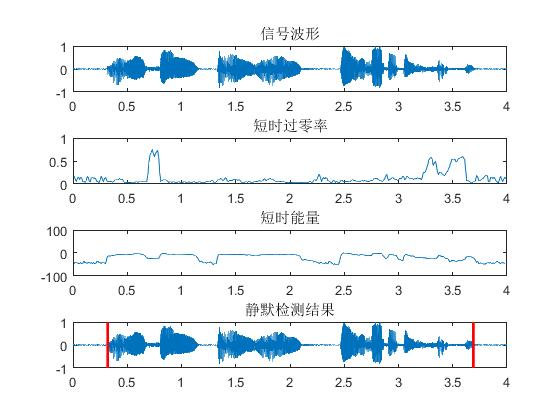展开全文matlab
• 短时能量主要用于区分浊音段和清音段，因为浊音E(i)值比清音时大得多；区分声母与韵母的分界和无话段与有话段分界。 计算第i帧语音信号yi(n)的短时能量公式为： E(i)=∑n=0L−1yi2(n),1<=i<=fn E(i)=\sum...
python语音信号处理（二）
一、短时能量
短时能量主要用于区分浊音段和清音段，因为浊音时E(i)值比清音时大得多；区分声母与韵母的分界和无话段与有话段分界。
计算第i帧语音信号yi(n)的短时能量公式为：
$E(i)=\sum_{n=0}^{L-1}y_i^2(n),\qquad1<=i<=fn$
求一帧语音的短时能量，直接上代码：
import numpy as np
import wave
import matplotlib.pyplot as plt
wlen=512
inc=128
f = wave.open(r"lantian.wav", "rb")
params = f.getparams()
nchannels, sampwidth, framerate, nframes = params[:4]
#print(str_data[:10])
wave_data = np.fromstring(str_data, dtype=np.short)
#print(wave_data[:10])
wave_data = wave_data*1.0/(max(abs(wave_data)))
print(wave_data[:10])
time = np.arange(0, wlen) * (1.0 / framerate)
signal_length=len(wave_data) #信号总长度
if signal_length<=wlen: #若信号长度小于一个帧的长度，则帧数定义为1
nf=1
else: #否则，计算帧的总长度
nf=int(np.ceil((1.0*signal_length-wlen+inc)/inc))
indices=np.tile(np.arange(0,wlen),(nf,1))+np.tile(np.arange(0,nf*inc,inc),(wlen,1)).T  #相当于对所有帧的时间点进行抽取，得到nf*nw长度的矩阵
print(indices[:2])
indices=np.array(indices,dtype=np.int32) #将indices转化为矩阵
a=frames[30:31]
print(a)
windown=np.hanning(512)
b=a*windown
c=np.square(b)
plt.figure(figsize=(10,4))
plt.plot(time,c,c="g")
plt.grid()
plt.show()

输出结果：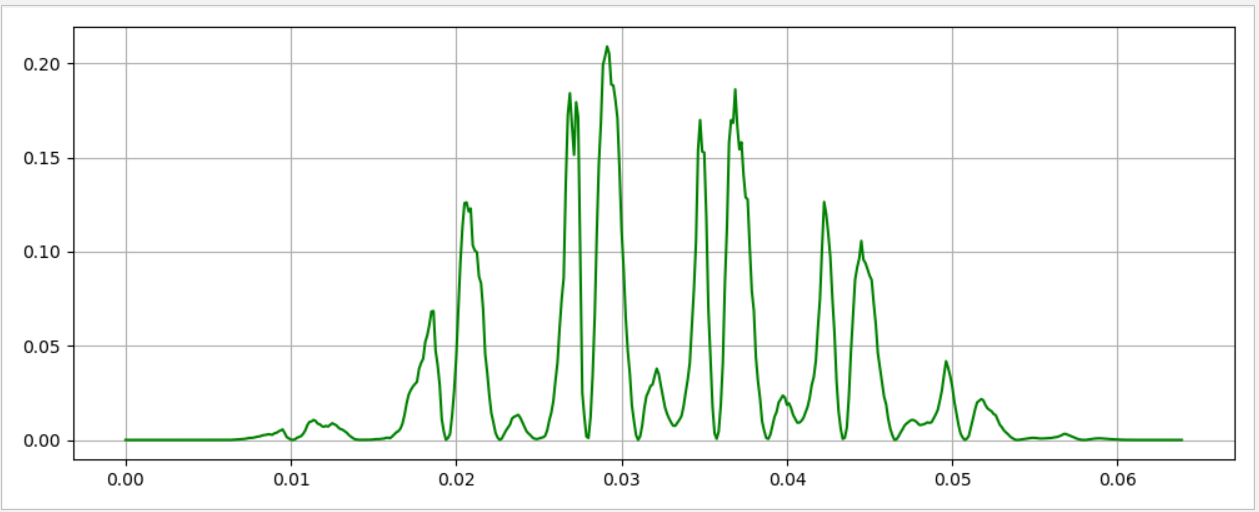信号加的是汉宁窗，上图为一帧语音的短时能量，下面为整段语音信号的短时能量。
import numpy as np
import wave
import matplotlib.pyplot as plt
wlen=512
inc=128
f = wave.open(r"lantian.wav", "rb")
params = f.getparams()
nchannels, sampwidth, framerate, nframes = params[:4]
wave_data = np.fromstring(str_data, dtype=np.short)
wave_data = wave_data*1.0/(max(abs(wave_data)))
print(wave_data[:10])
signal_length=len(wave_data) #信号总长度
if signal_length<=wlen: #若信号长度小于一个帧的长度，则帧数定义为1
nf=1
else: #否则，计算帧的总长度
nf=int(np.ceil((1.0*signal_length-wlen+inc)/inc))
print(nf)
indices=np.tile(np.arange(0,wlen),(nf,1))+np.tile(np.arange(0,nf*inc,inc),(wlen,1)).T  #相当于对所有帧的时间点进行抽取，得到nf*nw长度的矩阵
print(indices[:2])
indices=np.array(indices,dtype=np.int32) #将indices转化为矩阵
windown=np.hanning(wlen)
d=np.zeros(nf)
x=np.zeros(nf)
time = np.arange(0,nf) * (inc*1.0/framerate)
for i in range(0,nf):
a=frames[i:i+1]
b = a * windown
c=np.square(b)
d[i]=np.sum(c)
d = d*1.0/(max(abs(d)))
print(d)
plt.figure(figsize=(10,4))
plt.plot(time,d,c="g")
plt.grid()
plt.show()

输出结果为：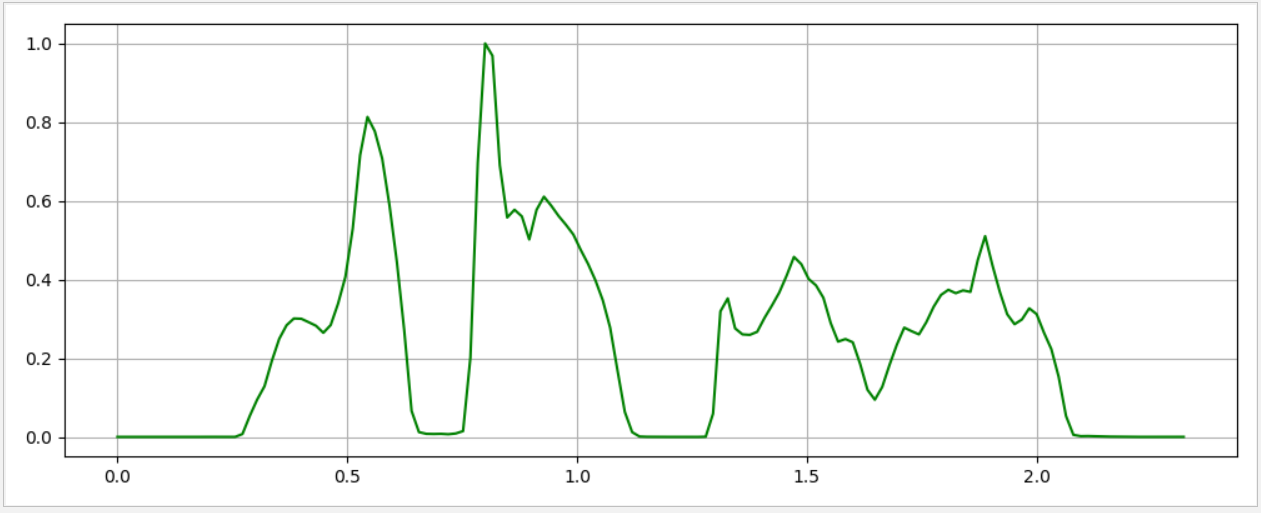横轴为时间，纵坐标为归一化后的短时能量。
该语音信号为普通话的“蓝天，白云”，可以比较清晰的看出短时能量的四个部分。
清音和浊音的区别：发清音时声带不振动，发浊音时声带振动。
二、短时平均过零率
​		短时平均过零率表示一帧语音中语音信号波形穿过横轴(零电平)的次数。过零率分析是.语音时域分析中最简单的一种。对于连续语音信号,过零即意味着时域波形通过时间轴;而对于离散信号,如果相邻的取样值改变符号,则称为过零。短时平均过零率就是样本数值改变符号的次数。
定义语音信号x(n)分帧后有yi(n),帧长为L，短时平均过零率为
$Z(i)=\frac{1}{2}\sum_{n=0}^{L-1}|sgn[y_{i}(n)]-sgn[y_i(n-1)]|,\qquad1<=i<=fn$
$sgn[n]=\begin{cases} -1，x>=0\\ 1， u<0\end{cases}$
上代码：
import numpy as np
import wave
wlen=512
inc=128
import matplotlib.pyplot as plt
f = wave.open(r"lantian.wav", "rb")
params = f.getparams()
nchannels, sampwidth, framerate, nframes = params[:4]
wave_data = np.fromstring(str_data, dtype=np.short)
wave_data = wave_data*1.0/(max(abs(wave_data)))
signal_length=len(wave_data) #信号总长度
if signal_length<=wlen: #若信号长度小于一个帧的长度，则帧数定义为1
nf=1
else: #否则，计算帧的总长度
nf=int(np.ceil((1.0*signal_length-wlen+inc)/inc))
indices=np.tile(np.arange(0,wlen),(nf,1))+np.tile(np.arange(0,nf*inc,inc),(wlen,1)).T  #相当于对所有帧的时间点进行抽取，得到nf*nw长度的矩阵
print(indices[:2])
indices=np.array(indices,dtype=np.int32) #将indices转化为矩阵
windown=np.hanning(wlen)
c=np.zeros(nf)
for i in range(nf):
a=frames[i:i+1]
b=windown*a
for j in range(wlen-1):
if b[j]*b[j+1]<0:
c[i]=c[i]+1
time = np.arange(0,nf) * (inc*1.0/framerate)
plt.figure(figsize=(10,4))
plt.plot(time,c,c="g")
plt.grid()
plt.show()

输出结果：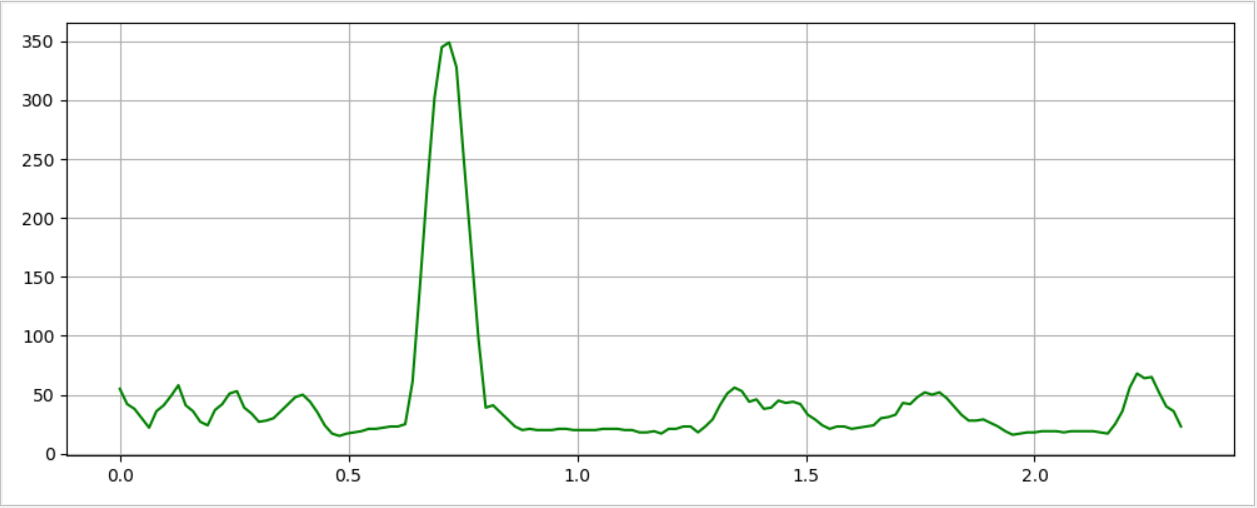​		通过分析语音信号发现，发浊音时，尽管声道有若干共振峰,但由于声门波引起谱的高频跌落,所以其语音能量约集中在3 kHz以下;而发清音时，多数能量出现在较高频率上。因为高频意味着高的短时平均过零率，低频意味着低的平均过零率，所以可以认为,浊音时具有较低的过零率，而清音时具有较高的过零率。当然,这种高低仅是相对而言的,并没有精确的数值关系。
​		利用短时平均过零率还可以从背景噪声中找出语音信号,可用于判断寂静无话段与有话段的起点和终点位置。在背景噪声较小时,用平均能量识别较为有效;而在背景噪声较大时,用短时平均过零率识别较为有效。
​		我们主要应用短时平均过零率来判断清音和浊音，有话段和无话段。
三、语音信号频域处理
​		在语音信号处理中，信号在频域或其他变换上的分析和处理占有重要地位，在频域和其他变换域上研究语音信号，可以使信号在时域上无法表现出来的某些特征变得十分明显。
下面将“蓝天，白云”这段语音信号转化为频域
import numpy as np
import wave
import matplotlib.pyplot as plt
f = wave.open(r"lantian.wav", "rb")
params = f.getparams()
nchannels, sampwidth, framerate, nframes = params[:4]
wave_data = np.fromstring(str_data, dtype=np.short)
wave_data = wave_data*1.0/(max(abs(wave_data)))
fft_signal = np.fft.fft(wave_data)     #语音信号FFT变换
fft_signal = abs(fft_signal)#取变换结果的模
plt.figure(figsize=(10,4))
time=np.arange(0,nframes)*framerate/nframes
plt.plot(time,fft_signal,c="g")
plt.grid()
plt.show()

输出结果：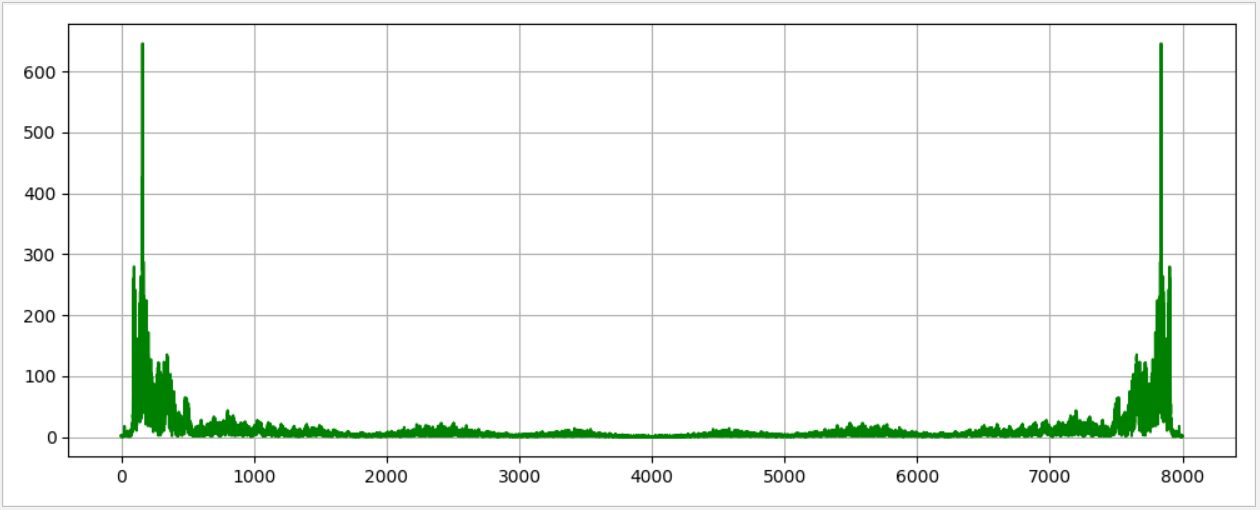短时傅里叶变换
​		在信号处理发展史上,每一次理论上的突破都带来了信号处理领域的重大变革。传统傅里叶变换( Fourier Transform,FT)是以应用数学为基础建立起来的一门学科,它将信号分解为各个不同频率分量的组合，使信号的时域特征与频域特征联系起来,成为信号处理的有力工具。但是傅里叶变换使用的是一种全局变换,因此它无法表述信号的时频局域性质。为了能够分析处理非平稳信号,人们对傅里叶变换进行了推广，提出了短时傅里叶变换(Short Time Fou-rier Transform, STFT)和其他变换域上的处理,这些理论都可应用于语音信号分析处理。
​		短时傅里叶分析(Short Time Fourier Analysis, STFA)适用于分析缓慢时变信号的频谱分析,在语音分析处理中已经得到广泛应用。其方法是先将语音信号分帧,再将各帧进行傅里叶变换。每一帧语音信号可以被认为是从各个不同的平稳信号波形中截取出来的,各帧语音的短时频谱就是各个平稳信号波形频谱的近似。
由于语音信号是短时平稳的，因此可以·对·语音·进行·分帧处理，计算某一帧的傅里叶变换，这样得到的就是短时傅里叶变换，其定义为
$X_n(e^{jw})=\sum_{m=-\infty}^{\infty}x(m)w(n-m)e^{jw}$
式中，下x(n)为语音信号序列：w(n)为实数窗序列，短时傅里叶变换是时间n和角频率w的函数，他反应了语音信号的频率随时间变化的特性。
[外链图片转存失败,源站可能有防盗链机制,建议将图片保存下来直接上传(img-RbV9gnE9-1573277367864)(C:\Users\jh\AppData\Roaming\Typora\typora-user-images\1572942290950.png)]
短时傅里叶变换有两种不同的解释，一种是当n固定不变时，X（ejw）为序列w(n-m)x(m)的标准傅里叶变换，此时X（ejw）具有与标准傅里叶变换相同的性质。另一种是当w固定不变时，可以将X（ejw）视为信号x(n)与窗函数指数加权w(n)ejw的卷积。
下面取语音的第50帧的语音信号做FFT变换：
import numpy as np
import wave
import matplotlib.pyplot as plt
wlen=512
inc=128
f = wave.open(r"lantian.wav", "rb")
params = f.getparams()
nchannels, sampwidth, framerate, nframes = params[:4]
wave_data = np.fromstring(str_data, dtype=np.short)
wave_data = wave_data*1.0/(max(abs(wave_data)))
print(wave_data[:10])
signal_length=len(wave_data) #信号总长度
if signal_length<=wlen: #若信号长度小于一个帧的长度，则帧数定义为1
nf=1
else: #否则，计算帧的总长度
nf=int(np.ceil((1.0*signal_length-wlen+inc)/inc))
print(nf)
indices=np.tile(np.arange(0,wlen),(nf,1))+np.tile(np.arange(0,nf*inc,inc),(wlen,1)).T  #相当于对所有帧的时间点进行抽取，得到nf*nw长度的矩阵
print(indices[:2])
indices=np.array(indices,dtype=np.int32) #将indices转化为矩阵
windown=np.hanning(wlen)
a=frames[50:51]
b=a*windown
fft_signal = np.fft.fft(b)
fft_signal=abs(fft_signal)
time=np.arange(0,wlen)*framerate/nframes
plt.figure(figsize=(10,4))
plt.plot(time,fft_signal,c="g")
plt.grid()
plt.show()

输出结果：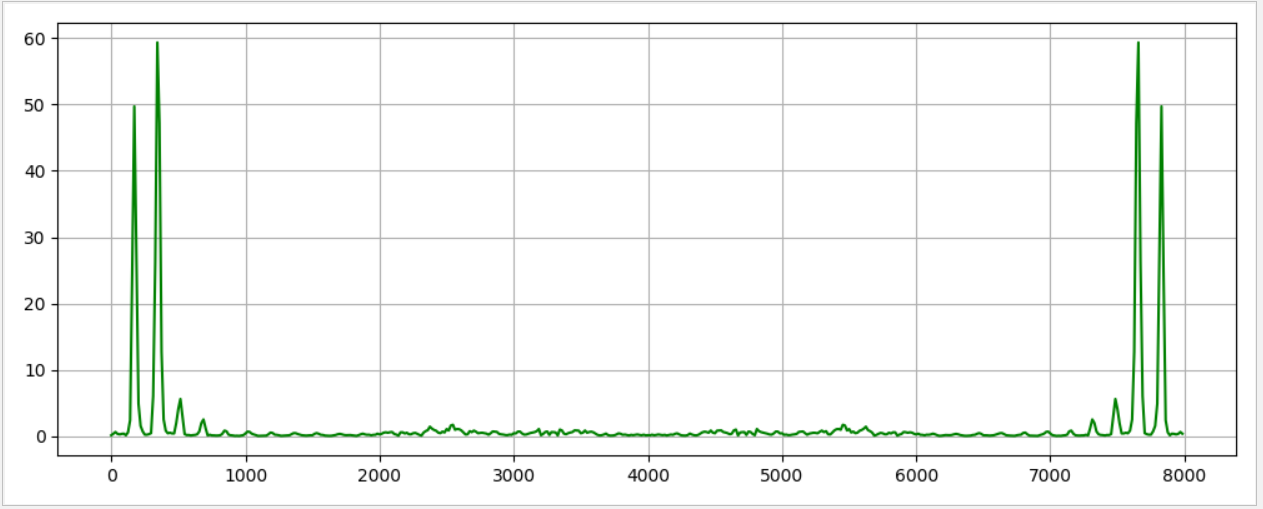语普图
​		通过语音的短时傅里叶分析可以研究语音的短时频谱随时间的变化关系。在数字信号处理(Digital Signal Processing ,DSP)技术发展起来以前,人们就已经利用语谱仪来分析和记录语音信号的短时频谱。语谱仪是把语音的电信号送人一-组频率依次相接的窄带滤波器中,各个窄带滤波器的输出经整流均方后按频率由低到高的顺序记录在一卷记录纸上。信号的强弱由记录在纸上的灰度来表示:如果某个滤波器输出的信号强,相应的记录将浓黑;反之,则浅淡一些。记录纸按照一定的速度旋转,相当于按不同的时间记录了相应的滤波器输出。由此得到的图形就是语音信号的语谱图,其水平方向是时间轴,垂直方向是频率轴,图上的灰度条纹代表各个时刻的语音短时谱。语谱图反映了语音信号的动态频谱特性,在语音分析中具有重要的实用价值,被称为可视语音。
import numpy as np
import wave
import matplotlib.pyplot as plt
f = wave.open(r"lantian.wav", "rb")
params = f.getparams()
nchannels, sampwidth, framerate, nframes = params[:4]
wave_data = np.fromstring(str_data, dtype=np.short)
wave_data = wave_data*1.0/(max(abs(wave_data)))
plt.specgram(wave_data,Fs = framerate, scale_by_freq = True, sides = 'default')
plt.show()

specgram()官网描述是：
matplotlib.pyplot.specgram(x, NFFT=None, Fs=None, Fc=None, detrend=None, window=None, noverlap=None, cmap=None, xextent=None, pad_to=None, sides=None, scale_by_freq=None, mode=None, scale=None, vmin=None, vmax=None, *, data=None, **kwargs)
参数：
x : 1-D array or sequence 数组或序列
Array or sequence containing the data.
数组或序列包含的数据。
Fs : scalar 标量
The sampling frequency (samples per time unit). It is used to calculate  the Fourier frequencies, freqs, in cycles per time unit. The default  value is 2.
抽样频率（每秒抽样数），用来计算傅里叶频率，以周期/时间单位表示。默认值为2
window : callable or ndarray 可调用的或无期限的
A function or a vector of length NFFT. To create window vectors see  window_hanning, window_none, numpy.blackman, numpy.hamming,  numpy.bartlett, scipy.signal, scipy.signal.get_window, etc. The default  is window_hanning. If a function is passed as the argument, it must take  a data segment as an argument and return the windowed version of the  segment.
长度为nfft的函数或向量。要创建窗口向量，请参见window_hanning、window_none、numpy.blackman、numpy.hamming、numpy.bartlett、scipy.signal、scipy.signal.get_window等。默认值为window_hanning。如果函数作为参数传递，则必须将数据段作为参数，并返回该段的窗口版本。
sides : {‘default’, ‘onesided’, ‘twosided’}
Specifies which sides of the spectrum to return. Default gives the  default behavior, which returns one-sided for real data and both for  complex data. ‘onesided’ forces the return of a one-sided spectrum,  while ‘twosided’ forces two-sided.
指定要返回频谱的哪一侧。默认值提供默认行为，对于实际数据和复杂数据都返回单面。单侧的力是单侧谱的返回，而“双侧的”力是双侧谱的返回。
The number of points to which the data segment is padded when performing  the FFT. This can be different from NFFT, which specifies the number of  data points used. While not increasing the actual resolution of the  spectrum (the minimum distance between resolvable peaks), this can give  more points in the plot, allowing for more detail. This corresponds to  the n parameter in the call to fft(). The default is None, which sets  pad_to equal to NFFT
NFFT : int
The number of data points used in each block for the FFT. A power 2 is  most efficient. The default value is 256. This should NOT be used to get  zero padding, or the scaling of the result will be incorrect. Use  pad_to for this instead.
detrend : {‘none’, ‘mean’, ‘linear’} or callable, default ‘none’
The function applied to each segment before fft-ing, designed to remove  the mean or linear trend. Unlike in MATLAB, where the detrend parameter  is a vector, in Matplotlib is it a function. The mlab module defines  detrend_none, detrend_mean, and detrend_linear, but you can use a custom  function as well. You can also use a string to choose one of the  functions: ‘none’ calls detrend_none. ‘mean’ calls detrend_mean.  ‘linear’ calls detrend_linear.
在FFT之前应用到每个段的函数，其被设计为去除平均值或线性趋势。与Matlab不同的是，dTrend参数是矢量，matplottlib是函数。MLAB模块定义DETrend_None、DeTrend_mean和DeTrend_Linear，但也可以使用自定义函数。您还可以使用字符串来选择其中一个函数：“无”调用deTrend_none。“mean”调用dTrend_mean。“线性”调用dTrend_linear。
scale_by_freq : bool, optional
Specifies whether the resulting density values should be scaled by the  scaling frequency, which gives density in units of Hz^-1. This allows  for integration over the returned frequency values. The default is True  for MATLAB compatibility.
指定所产生的密度值是否应通过缩放频率进行缩放，该频率提供以Hz^-1为单位的密度。这允许对返回的频率值进行集成。默认情况下，MATLAB兼容性是正确的
mode : {‘default’, ‘psd’, ‘magnitude’, ‘angle’, ‘phase’}
What sort of spectrum to use. Default is ‘psd’, which takes the power  spectral density. ‘magnitude’ returns the magnitude spectrum. ‘angle’  returns the phase spectrum without unwrapping. ‘phase’ returns the phase  spectrum with unwrapping.
使用什么样的频谱。默认值是“psd”，它采用功率谱密度。Magnition“返回幅度谱。”Angle“返回相位谱而不展开。”“phase”返回展开后的相位谱。
noverlap : int
The number of points of overlap between blocks. The default value is 128.
块之间重叠点的数目。默认值是128。
scale : {‘default’, ‘linear’, ‘dB’}
The scaling of the values in the spec. ‘linear’ is no scaling. ‘dB’  returns the values in dB scale. When mode is ‘psd’, this is dB power (10  * log10). Otherwise this is dB amplitude (20 * log10). ‘default’ is  ‘dB’ if mode is ‘psd’ or ‘magnitude’ and ‘linear’ otherwise. This must  be ‘linear’ if mode is ‘angle’ or ‘phase’.
规范中值的缩放。“线性”不是缩放。‘db’返回db比例尺中的值。当模式为‘PSD’时，这是db功率(10log 10)。否则，这是db振幅(20log 10)。默认的是‘db’，如果模式是‘PSD’或‘大小’，‘线性’，否则。这必须是‘线性’，如果模式是‘角度’或‘相位’。
Fc : int
The center frequency of x (defaults to 0), which offsets the x extents  of the plot to reflect the frequency range used when a signal is  acquired and then filtered and downsampled to baseband.
x的中心频率(默认值为0)，它抵消了图中的x个范围，以反映在获取信号、然后滤波和降采样到基带时所使用的频率范围。
cmap
A matplotlib.colors.Colormap instance; if None, use default determined by rc
matplotlib.chros.colmap实例；如果没有，则使用rc确定的默认值。
xextent : None or (xmin, xmax)
The image extent along the x-axis. The default sets xmin to the left  border of the first bin (spectrum column) and xmax to the right border  of the last bin. Note that for noverlap>0 the width of the bins is  smaller than those of the segments.
沿x轴的图像范围。默认值为第一个bin（频谱列）的左边界和最后一个bin的右边界的xmax。请注意，对于NoVerlap>0，bin的宽度小于段的宽度。
**kwargs
Additional kwargs are passed on to imshow which makes the specgram image.
更多的kwargs被传递到图像中，这就产生了散斑图像。
返回值：
spectrum : 2-D array
Columns are the periodograms of successive segments.
列是连续段的周期图
freqs : 1-D array
The frequencies corresponding to the rows in spectrum.
与光谱中的行相对应的频率。
t : 1-D array
The times corresponding to midpoints of segments (i.e., the columns in spectrum).
对应于分段中点(即频谱中的列)的时间。
im : instance of class AxesImage
The image created by imshow containing the spectrogram
包含频谱图的图像
Notes
The parameters detrend and scale_by_freq do only apply when mode is set to ‘psd’.
只有当“模式”设置为“PSD”时，参数“按频率递减和缩放”才适用。
Note
In addition to the above described arguments, this function can take a  data keyword argument. If such a data argument is given, the following  arguments are replaced by data[]:
All arguments with the following names: ‘x’.
Objects passed as data must support item access (data[]) and membership test ( in data).
返回：
spectrum:频谱矩阵
freqs：频谱图每行对应的频率
ts：频谱图每列对应的时间
fig ：图像
输出结果：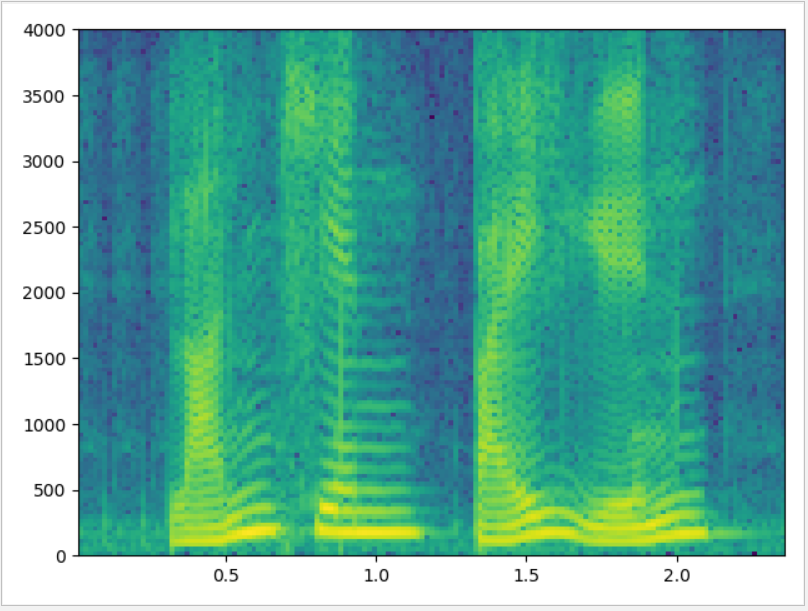​		语谱图的横坐标是时间，纵坐标是频率，坐标点值为语音数据能量。由于是采用二维平面表达三维信息，所以能量值的大小是通过颜色来表示的，颜色深，表示该点的语音能量越强。

​		我们可以观察语音不同频段的信号强度随时间的变化情况。由于音乐信号本身频率丰富，不太容易看出规律，我们可以观察一下纯粹的语音数据的语谱图。从图中可以看到明显的一条条横方向的条纹，我们称为“声纹”，有很多应用。条纹的地方实际是颜色深的点聚集的地方，随时间延续，就延长成条纹，也就是表示语音中频率值为该点横坐标值的能量较强，在整个语音中所占比重大，那么相应影响人感知的效果要强烈得多。而一般语音中数据是周期性的，所以，能量强点的频率分布是频率周期的，即存在300Hz强点，则一般在n*300Hz点也会出现强点，所以我们看到的语谱图都是条纹状的。尽管客观人发声器官的音域是有限度的，即一般人发声最高频率为4000Hz，乐器的音域要比人宽很多，打击乐器的上限可以到20KHz。但是，由于我们数字分析频率时，采用的是算法实现的，一般是FFT，所以其结果是由采样率决定的，即尽管是上限为4000Hz的语音数据，如果采用16Khz的采样率来分析，则仍然可以在4000Hz以上的频段发现有数据分布，则可以认为是算法误差，非客观事实

语谱图生成流程（引用）
引用blog:
语音信号处理之（四）梅尔频率倒谱系数（MFCC）
我们处理的是语音信号，那么如何去描述它很重要。因为不同的描述方式放映它不同的信息。那怎样的描述方式才利于我们观测，利于我们理解呢？这里我们先来了解一个叫声谱图的东西。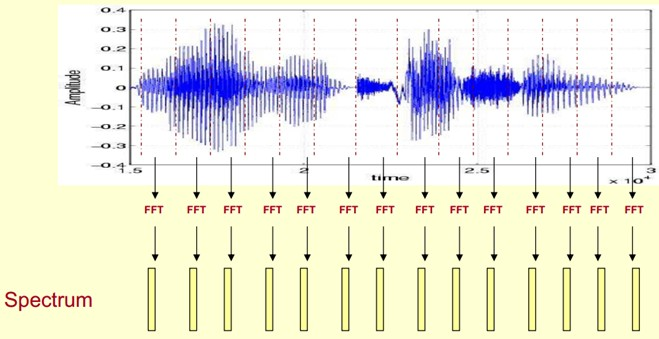​		这里，这段语音被分为很多帧，每帧语音都对应于一个频谱（通过短时FFT计算），频谱表示频率与能量的关系。在实际使用中，频谱图有三种，即线性振幅谱、对数振幅谱、自功率谱（对数振幅谱中各谱线的振幅都作了对数计算，所以其纵坐标的单位是dB（分贝）。这个变换的目的是使那些振幅较低的成分相对高振幅成分得以拉高，以便观察掩盖在低幅噪声中的周期信号）。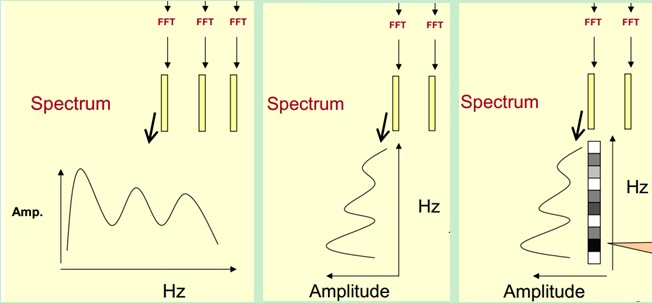​		我们先将其中一帧语音的频谱通过坐标表示出来，如上图左。现在我们将左边的频谱旋转90度。得到中间的图。然后把这些幅度映射到一个灰度级表示（也可以理解为将连续的幅度量化为256个量化值？），0表示黑，255表示白色。幅度值越大，相应的区域越黑。这样就得到了最右边的图。那为什么要这样呢？为的是增加时间这个维度，这样就可以显示一段语音而不是一帧语音的频谱，而且可以直观的看到静态和动态的信息。优点稍后呈上。
​	 	这样我们会得到一个随着时间变化的频谱图，这个就是描述语音信号的spectrogram声谱图。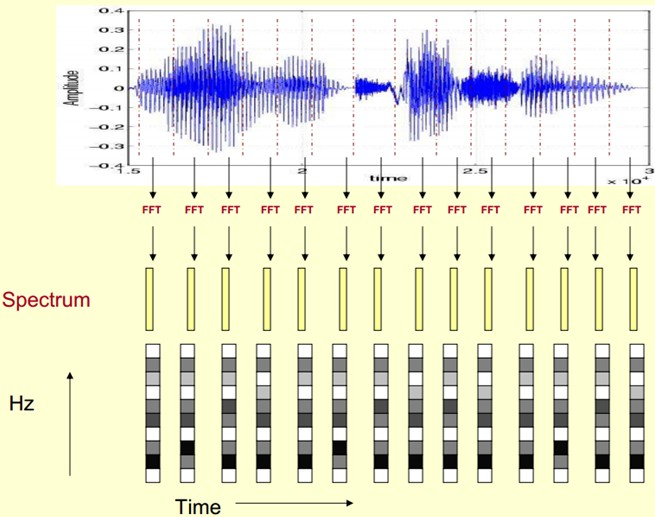下图是一段语音的声谱图，很黑的地方就是频谱图中的峰值（共振峰formants）。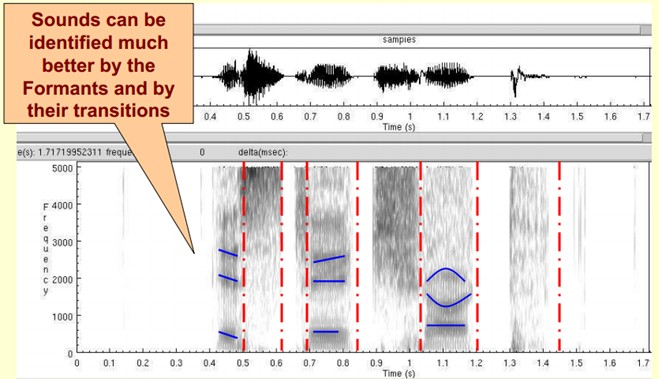那我们为什么要在声谱图中表示语音呢？
​		首先，音素（Phones）的属性可以更好的在这里面观察出来。另外，通过观察共振峰和它们的转变可以更好的识别声音。隐马尔科夫模型（Hidden Markov Models）就是隐含地对声谱图进行建模以达到好的识别性能。还有一个作用就是它可以直观的评估TTS系统（text to speech）的好坏，直接对比合成的语音和自然的语音声谱图的匹配度即可。
研究加不同长度的窗函数对语谱图的影响
​		语谱图的时间分辨率和频率分辨率是由窗函数的特性决定的，可以按照短时傅里叶变换的第一种解释来分析频率分辨率。如果需要观察语音谐波的细节，则需要提高语谱图的频率分辨率，也就是减小窗函数的带通宽度。由于带通宽度是与窗函数成反比的，因此提高频率分辨率必须要增加窗长。这种情况下得到的语谱图称为窄带语谱图。
实际上就是信号的时宽越大（小），信号的频带宽度越小（大）
窄带语谱图
依然是上面的“蓝天，白云”的语音，语音采样频率为8000HZ，取窗长为512个数据点，帧移为窗长的1/4，即128个数据点。
import numpy as np
import wave
import matplotlib.pyplot as plt
def windows(name='Hamming', N=20):
# Rect/Hanning/Hamming
if name == 'Hamming':
window = np.array([0.54 - 0.46 * np.cos(2 * np.pi * n / (N - 1)) for n in range(N)])
elif name == 'Hanning':
window = np.array([0.5 - 0.5 * np.cos(2 * np.pi * n / (N - 1)) for n in range(N)])
elif name == 'Rect':
window = np.ones(N)
return window
f = wave.open(r"lantian.wav", "rb")
params = f.getparams()
nchannels, sampwidth, framerate, nframes = params[:4]
wave_data = np.fromstring(str_data, dtype=np.short)
wave_data = wave_data*1.0/(max(abs(wave_data)))
plt.specgram(wave_data,Fs = framerate,NFFT=512,window=windows("Hanning",512),noverlap=384,scale_by_freq = True, sides = 'default')
plt.show()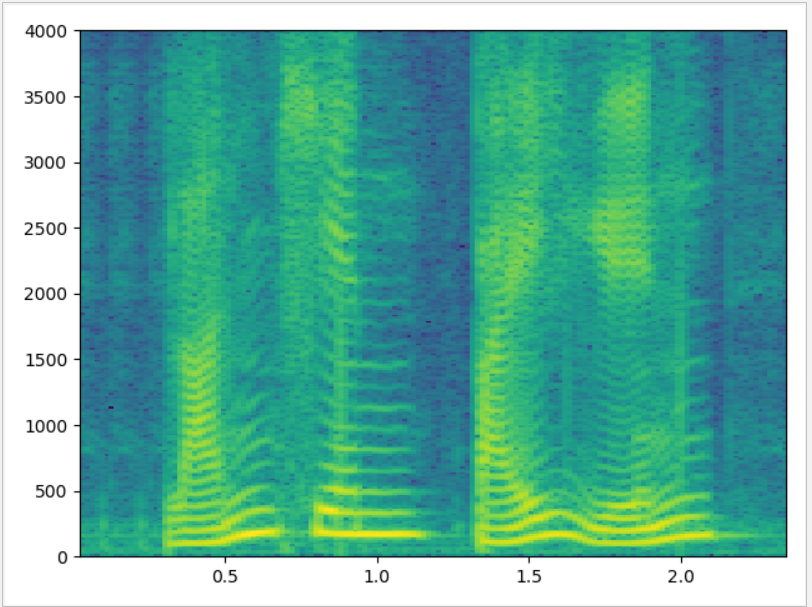从结果图中可以清楚看到谐波的结构，频率分辨率非常好，但是时间上的分辨率就不理想.
窄带语谱图，频率分辨率太过精细，不能很好体现出共振峰的大致位置，即反映不出基波的变化特性。
宽带语谱图
依然是上面的“蓝天，白云”的语音，语音采样频率为8000HZ，取窗长为128个数据点，帧移为窗长的1/4，即32个数据点.
import numpy as np
import wave
import matplotlib.pyplot as plt
def windows(name='Hamming', N=20):
# Rect/Hanning/Hamming
if name == 'Hamming':
window = np.array([0.54 - 0.46 * np.cos(2 * np.pi * n / (N - 1)) for n in range(N)])
elif name == 'Hanning':
window = np.array([0.5 - 0.5 * np.cos(2 * np.pi * n / (N - 1)) for n in range(N)])
elif name == 'Rect':
window = np.ones(N)
return window
f = wave.open(r"lantian.wav", "rb")
params = f.getparams()
nchannels, sampwidth, framerate, nframes = params[:4]
wave_data = np.fromstring(str_data, dtype=np.short)
wave_data = wave_data*1.0/(max(abs(wave_data)))
plt.specgram(wave_data,Fs = framerate,NFFT=128,window=windows("Hanning",128),noverlap=96,scale_by_freq = True, sides = 'default')
plt.show()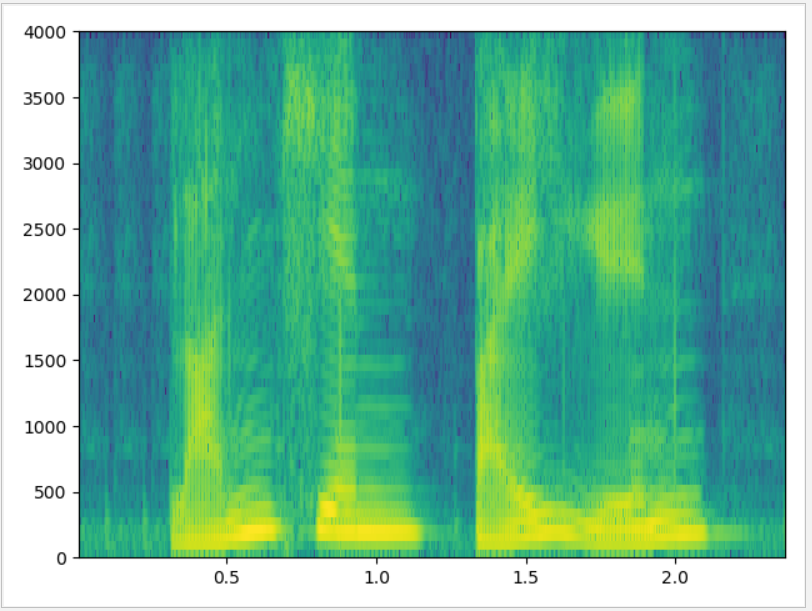​		与窄带语谱图相反，宽带语谱图的时间分辨率很好，频率分辨率较低，不能很好反映声音的纹理特性，反映了频谱的时变特性，能很好分辨出共振峰的大致位置，但分辨不清谐波结构。
研究加不同窗对语谱图的影响
import numpy as np
import wave
import matplotlib.pyplot as plt
def windows(name='Hamming', N=20):
# Rect/Hanning/Hamming
if name == 'Hamming':
window = np.array([0.54 - 0.46 * np.cos(2 * np.pi * n / (N - 1)) for n in range(N)])
elif name == 'Hanning':
window = np.array([0.5 - 0.5 * np.cos(2 * np.pi * n / (N - 1)) for n in range(N)])
elif name == 'Rect':
window = np.ones(N)
return window
f = wave.open(r"lantian.wav", "rb")
params = f.getparams()
nchannels, sampwidth, framerate, nframes = params[:4]
wave_data = np.fromstring(str_data, dtype=np.short)
wave_data = wave_data*1.0/(max(abs(wave_data)))
plt.specgram(wave_data,Fs = framerate, window=windows("Hanning",256),scale_by_freq = True, sides = 'default')
plt.show()

加海l宁窗的效果图：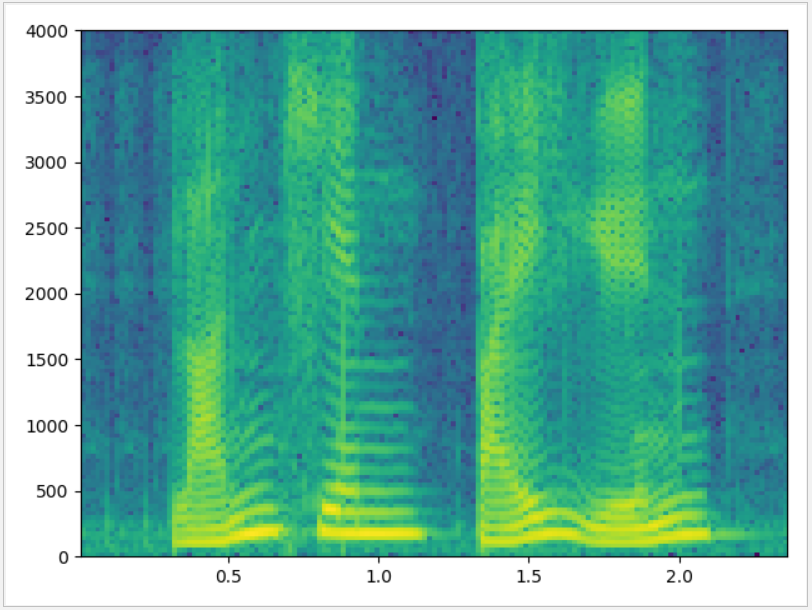加汉明窗的效果图：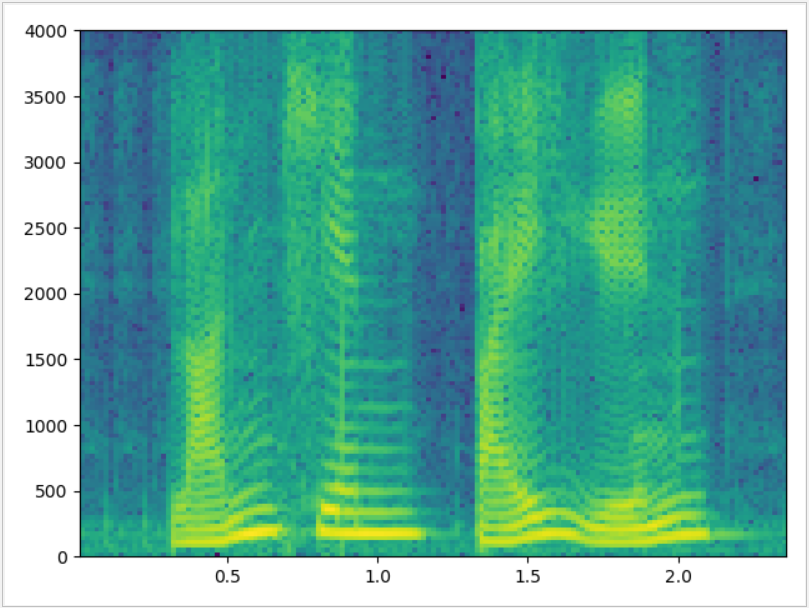加矩形窗的效果图：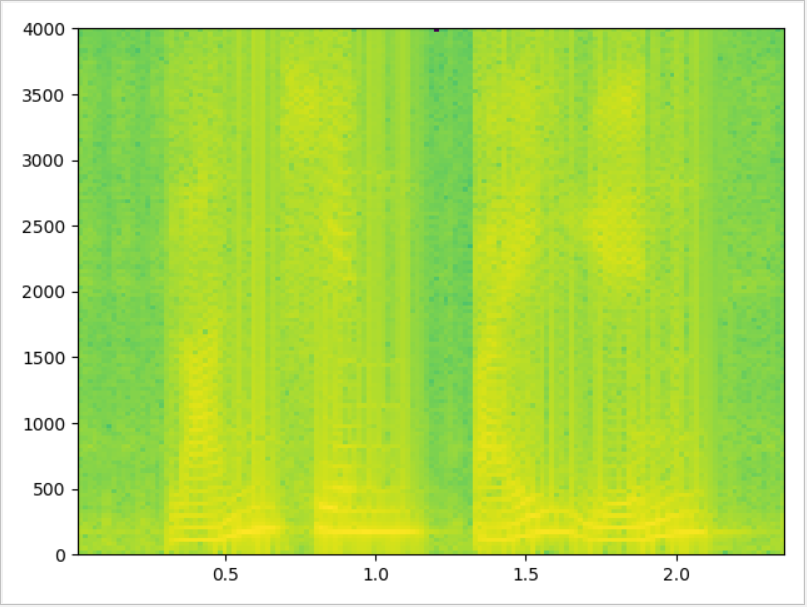写的比较乱，哪里有错误还请大佬们指正。


展开全文• 计算每一帧的短时能量，得到语音的短时能量 计算每一帧语音的过零数，得到短时帧过零数 考擦语音的平均能量设置一个较高的门限T1，用以确定语音的开始，然后根据背景噪声的平均能量确定一个稍低的门限T2，用以确定...
• 短时能量简介 语音信号是随时间变化的非平稳随机过程，因此对于语音的分析一般都是短时分析。这是因为语音虽然是时变的但是具有短时相关性，这个相关性来源于人的发生器官具有惯性，因此语音的状态是不会发生突变，...
• 基于短时时域处理中短时能量和过零率的语音端点检测方法 1.背景 在语音信号处理中检测出语音的端点是相当重要的。语音端点的检测是指从包含语音的一段信号中确定的起始点和结束点位置，因为在某些语音特性检测和...
• python求语音信号语谱图、短时能量短时过零率 转自：链接: ...python 语音识别 自然语言处理
• 2.短时能量计算代码 clear all; clc; close all; filedir=[]; % 设置路径 filename='bluesky3.wav'; % 设置文件名 fle=[filedir filename]; % 构成完整的路径和文件名 [x,Fs]=audioread(fle); % 读入数据文件 wlen=...
• 基于短时能量的语音端点检测以用来提取有声段的mfcc参数 matlab的代码each信号处理 数字信号处理 matlab
• 使用Yaafe提取音频特征（MFCC、短时能量短时过零率等特征）前段时间装了Yaafe库，今天就使用Yaafe来提取音频特征。如果没安装Yaafe，请参考我之前的文章。 Yaafe安装教程 使用Yaafe提取音频特征MFCC短时能量短时...
• 提取语音特征（短时能量，平均幅度、平均过零率）的matlab代码语音特征提取
• 时幅度是使用了信号绝对值的加权和，解决了较大级别的信号能量值敏感的问题（能量有平方项），在图中，对比时能量和时幅度，在清音的部分差别很明显，但浊音区域和清音区域间的级别差异不如能量时明显。信号处理 数字信号处理
• 首先是基于短时能量和短视过零率的端点检测=〉各变换域=〉人工神经网络=〉基于倒谱距离的检测算法=〉基于谱熵的方法=〉几何门限的方法=〉sigma函数=〉近些年的从分形技术和混沌理论引入的端点检测。 作为最早的短时...
• 元音音频截取 一、需求分析 ...求出整段音频的能量，再将这段音频的每个点除以[Math Processing Error]a\sqrt{a}： [Math Processing Error]En=∑0Nx2 En=\sum\limits_{0}^{N}{{ x ^{2}}} ...音频 截取
• import wave import pyaudio import numpy as np import pylab ...# 计算每一帧的能量 256个采样点为一帧 def calEnergy(wave_data) : energy = [] sum = 0 for i in range(len(wave_data)) : ...
• 语音信号的典型特征包括短时能量分析，过零率，短时自相关系数（下一个博客再说）等。实现语音信号的短时分析，首先实现加窗分帧就要。 分帧 一般来说假定语音信号在10ms到30ms之间是一个平稳的信号，窗函数平滑的...语音信号处理 python
• 运行程序后求出的短时能量图如图所示： 附： 程序中调用了frame2time函数，用于计算分帧后每一帧对应的时间。具体代码如下： function frameTime = frame2time (frameNum,framelen,inc,fs) % 分帧后......